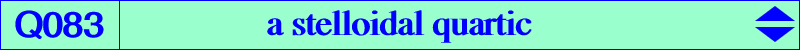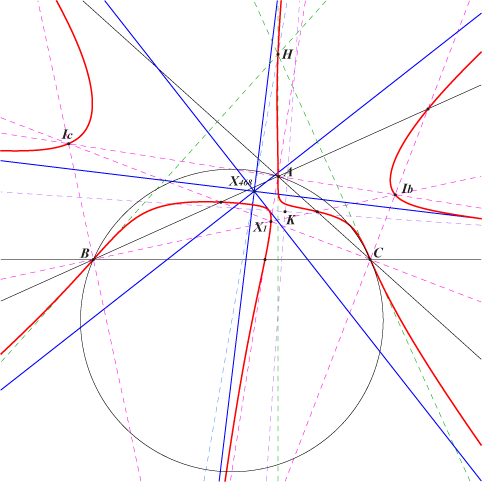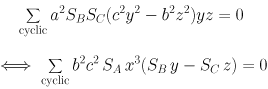X(1), X(4), X(2635) X(10221) and two other imaginary points on the Euler line, these three points also on K019, K511, Q083 excenters feet of bisectors extraversions of X(2635)Q083 is an example of stelloidal quartic i.e. a quartic with four real concurring asymptotes such that two consecutive asymptotes make an angle of 45°. These asymptotes concur at X(468), the intersection of the Euler line and the orthic axis. This point is called the radial center of the stelloid. This type of curve is also called a harmonic curve since its Laplacian identically vanishes. The polar line of any point on the McCay cubic (a stelloidal cubic) with respect to Q083 passes through H. This is the case of A, B, C (inflexion points), X(1), the excenters and naturally H. The polar conic of H is the Jerabek hyperbola (a stelloidal conic). These properties are actually true for any point M of the plane since the polar curves of any degree in a stelloid are themselves stelloids. A construction of Q083 is given in the page Q002. The isogonal transform of Q083 is Q084. See the related stelloidal cubic K598. Locus properties : Q083 is the locus of point P such that • P, its isogonal conjugate P* and O/P* are collinear. O/P* is the O-Ceva conjugate of P*. • equivalently, H, P and P*/P are collinear. See Q002, locus property 9, for other similar curves.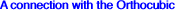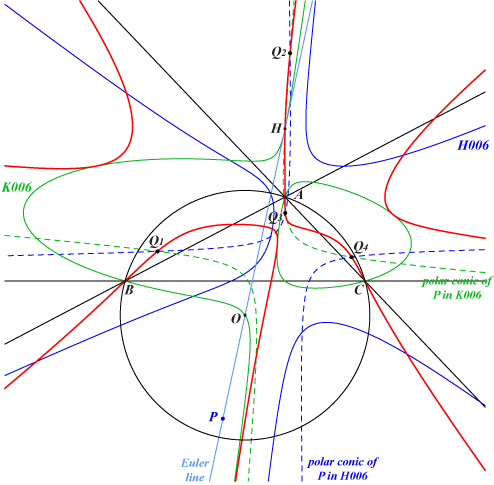The Orthocubic K006 and its hessian H006 share the same orthic line namely the Euler line. Thus, for any point P on the Euler line, the polar conics of P with respect to K006 and H006 are both rectangular hyperbolas intersecting at Q1, Q2, Q3, Q4. When P traverses the Euler line, the locus of these four points is the quartic Q083. Special cases : • with P = H, the hyperbolas are diagonal conics intersecting at the in/excenters. Note that the tangents at these points to Q083 and K006 coincide. • with P = O, the hyperbolas are circum-conics passing through H. *** See Q002, K100 for analogous properties when K006 is replaced with K003, K004 respectively.